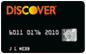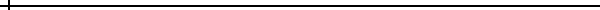Electric Wheelchair / Scooter Batteries

Please Call to Confirm Price & Availability

(Lead pricing is all over the place and we cannot guarantee posted pricing)

Call or E-Mail for Shipping Cost

Office: 603-425-9876

 Wheelchair / Scooter Description A-BEC, BEC 40, AGM AGM, 12 Volt, 33 Ah, L=7.7 x W=5.1 X H=6.5 inches, per battery, device may require (2) batteries A-BEC, Scoota, AGM AGM, 12 Volt, 33 Ah, L=7.7 x W=5.1 x H=6.5 inches, also fits the Scoota Plus, Scoota Bug Model A-BEC, Std. Series, AGM AGM, 12 Volt, 33 Ah, L=7.7 x W=5.1 x H=6.5 inches, Price is for one battery, device may require (2) packs A-BEC, Sterling, AGM AGM, 12 Volt, 33 Ah, L=7.7 x W=5.1 x H=6.5 inches, Price is for one battery, device may require (2) packs A-BEC, Targa Series, AGM AGM, 12 Volt, 33 Ah, L=7.7 x W=5.1 x H=6.5 inches, Price is for one battery, device may require (2) packs Adaptive Driving Systems Model 12 AGM Fits all Adaptive Driving Systems Model 12 Group 24, 12 Volt, 70 Ah, L=10.2 x W=6.6 x H=8.2 inches Adaptive Driving Systems Model 14 AGM AGM, 22NF, 12 Volt, 55 Ah, L=9.0 x W=5.4 x H=8.1 inches per battery, device requires 2 batteries Advanced Technology (All Models) AGM AGM, Group 24, 12 Volt, 70 Ah, L=10.2, W=6.6, H=8.2 inches, Device requires only one battery Alpha Unlimited (All Models) AGM AGM, Group 24, 12 Volt, 70 Ah, L=10.2, W=6.6, H=8.2 inches, Device requires (2) batteries AM Scooter (All Models) AGM AGM, 12 Volt, 33 Ah, L=7.7 x W=5.1 x H=6.5 inches, Price is for one battery, device may require (2) packs American Vermerien (All Models) AGM AGM, Group 24, 12 Volt, 70 Ah, L=10.2, W=6.6, H=8.2 inches, Device requires (2) batteries Amigo FD (Front Drive) AGM AGM, 12 Volt, 33 Ah, L=7.7 x W=5.1 x H=6.5 inches, Price is for one battery, Unit requires (1) Battery Braun Corp., T1100/1200F (Series 1-3) AGM AGM, Group 24, 12 Volt, 70 Ah, L=10.2, W=6.6, H=8.2 inches, Device requires (2) batteries Braun Corp., T1100/1200F (Series 4 and later) AGM, 12 Volt, 33 Ah, L=7.7 x W=5.1 x H=6.5 inches, Price is for one battery, device may require (2) packs Bruno Regal AGM AGM, 12 Volt, 33 Ah, L=7.7 x W=5.1 x H=6.5 inches, Price is for one battery, device may require (2) packs Carter Co., (All Models) AGM AGM, Group 24, 12 Volt, 70 Ah, L=10.2, W=6.6, H=8.2 inches, Device requires (2) batteries Damaco D90/D99 AGM, 12 Volt, 33 Ah, L=7.7 x W=5.1 x H=6.5 inches, Price is for one battery, device may require (2) packs Damaco Electro-Lite AGM AGM, 12 Volt, 33 Ah, L=7.7 x W=5.1 x H=6.5 inches, Price is for one battery, device may require (2) packs Damaco Elite AGM AGM, 12 Volt, 33 Ah, L=7.7 x W=5.1 x H=6.5 inches, Price is for one battery, device may require (2) packs Damaco Ovation AGM AGM, 12 Volt, 33 Ah, L=7.7 x W=5.1 x H=6.5 inches, Price is for one battery, device may require (2) packs Dignified Products (All Models) AGM AGM, 12 Volt, 33 Ah, L=7.7 x W=5.1 x H=6.5 inches, Price is for one battery EF Brewer Co., (All Models) AGM AGM, 12 Volt, 33 Ah, L=7.7 x W=5.1 x H=6.5 inches, Price is for one battery, One battery per unit Electric Mobility Butler AGM AGM, 12 Volt, 33 Ah, L=7.7 x W=5.1 x H=6.5 inches, Price is for one battery Electric Mobility Cycle Chair AGM AGM, 12 Volt, 33 Ah, L=7.7 x W=5.1 x H=6.5 inches, Price is for one battery Electric Mobility Rascal MWD Power Chair AGM AGM, 22NF, 12 Volt, 55 Ah, L=9.0, W=5.4, H=8.1 inches, price per battery, device requires (2) batteries Electric Mobility Rascal Power Chair AGM AGM, 22NF, 12 Volt, 55 Ah, L=9.0, W=5.4, H=8.1 inches, price per battery, device requires (2) batteries Electric Mobility Rascal Scooter AGM AGM, 12 Volt, 33 Ah, L=7.7 x W=5.1 x H=6.5 inches, Price is for one battery Electric Mobility Rover, Sparky, Squire models AGM AGM, Group 24, 12 Volt, 70 Ah, L=10.2, W=6.6, H=8.2 inches, Device requires (2) batteries Electric Mobility Via AGM AGM, 22NF, 12 Volt, 55 Ah, L=9.0, W=5.4, H=8.1 inches, price per battery, device requires (2) batteries Electric Wheelchair (All Models) AGM, 12 Volt, 33 Ah, L=7.7 x W=5.1 x H=6.5 inches, Price is for one battery, device requires (2) batteries Everest & Jennings Marathon (ES, ES-GT, LTD) Marathon AGM, 22NF, 12 Volt, 55 Ah, L=9.0, W=5.4, H=8.1 inches, price per battery, device requires (2) batteries Everest & Jennings 14 Belt Drive Chairs, Hot Wheels AGM, 12 Volt, 33 Ah, L=7.7 x W=5.1 x H=6.5 inches, Price is for one battery, device requires (2) batteries Everest & Jennings 3N, Tempest, Metro Power, Quest AGM, 12 Volt, 33 Ah, L=7.7 x W=5.1 x H=6.5 inches, Price is for one battery, device requires (2) batteries Everest & Jennings Explorer, Lancer, Vortex, Solaire models AGM, Group 24, 12 Volt, 70 Ah, L=10.2, W=6.6, H=8.2 inches, Device requires (2) batteries Everest & Jennings Magnum, Magnum Power Recliner AGM, 22NF, 12 Volt, 55 Ah, L=9.0, W=5.4, H=8.1 inches, price per battery, device requires (2) batteries Everest & Jennings Xcaliber, Xcaliber power, Sprint, Sprint power AGM, 22NF, 12 Volt, 55 Ah, L=9.0, W=5.4, H=8.1 inches, price per battery, device requires (2) batteries Falcon Rehab (All Models) AGM AGM Group 27, 12 Volt, 100 Ah., L=12.0, W=6.6, H=8.2 inches Per Battery, this device requires (2) Batteries Gaymar-Retec (All Models) GEL AGM Group 27, 12 Volt, 100 Ah., L=12.0, W=6.6, H=8.2 inches Per Battery, this device requires (2) Batteries Gendron-Solo (All Models)AGM AGM, 22NF, 12 Volt, 55 Ah, L=9.0, W=5.4, H=8.1 inches, price per battery, device requires (2) batteries Genus (All Models) AGM AGM, 12 Volt, 33 Ah, L=7.7 x W=5.1 x H=6.5 inches, Price is for one battery, device requires (2) batteries Global Research Galaxy, Starlight 1&2 models, AGM AGM, 12 Volt, 33 Ah, L=7.7 x W=5.1 x H=6.5 inches, Price is for one battery, this unit requires only one battery Global Research Starlight 3 AGM AGM, 12 Volt, 33 Ah, L=7.7 x W=5.1 x H=6.5 inches, Price is for one battery, device requires (2) batteries Golden Technology GSE 600/700/300/400 models AGM AGM, 12 Volt, 33 Ah, L=7.7 x W=5.1 x H=6.5 inches, Price is for one battery, device requires (2) batteries Golden Technology Sterling, Scoota, Scoota Bug, Hawk models AGM, 12 Volt, 33 Ah, L=7.7 x W=5.1 x H=6.5 inches, Price is for one battery, device requires (2) batteries Hoveround Activa DM, LX, Affinity, MPV, 2/3/4, HRV 100 model AGM, 12 Volt, 33 Ah, L=7.7 x W=5.1 x H=6.5 inches, Price is for one battery, device requires (2) batteries Hoveround Teknique HD AGM AGM, Group 24, 12 Volt, 70 Ah, L=10.2, W=6.6, H=8.2 inches, Device requires (2) batteries Hoveround Teknique RWD/FWD/GT models AGM AGM, 22NF, 12 Volt, 55 Ah, L=9.0, W=5.4, H=8.1 inches, price per battery, device requires (2) batteries Independence Chair (All Models) AGM AGM, 12 Volt, 33 Ah, L=7.7 x W=5.1 x H=6.5 inches, Price is for one battery, device requires (2) batteries Invacare Arrow XT, Strom Arrow models, AGM AGM, Group 24, 12 Volt, 70 Ah, L=10.2, W=6.6, H=8.2 inches, Device requires (2) batteries Invacare Arrow Ranger X/11, Power Tiger, Storm RX2, etc. AGM, 22NF, 12 Volt, 55 Ah, L=9.0, W=5.4, H=8.1 inches, price per battery, device requires (2) batteries Invacare Jaguar, Rabbit, Power 9000, (14" wide or less) AGM, 12 Volt, 33 Ah, L=7.7 x W=5.1 x H=6.5 inches, Price is for one battery, device requires (2) batteries Lakematic (All Models) AGM AGM, Group 24, 12 Volt, 70 Ah, L=10.2, W=6.6, H=8.2 inches, Device requires (2) batteries Leisure Lift / Pace Saver / Burke Mobility AGM, 12 Volt, 33 Ah, L=7.7 x W=5.1 x H=6.5 inches, Price is for one battery, device requires (1) or (2) batteries Medical Resource Co., (All Models) AGM AGM, 12 Volt, 33 Ah, L=7.7 x W=5.1 x H=6.5 inches, Price is for one battery, device requires (2) batteries Mobility Manufacturing Bobcat AGM AGM, 12 Volt, 33 Ah, L=7.7 x W=5.1 x H=6.5 inches, Price is for one battery, device requires (2) batteries Motovator (All Models) AGM AGM, 12 Volt, 33 Ah, L=7.7 x W=5.1 x H=6.5 inches, Price is for one battery, device requires (1) or (2) batteries Newton (All Models) AGM AGM, 12 Volt, 33 Ah, L=7.7 x W=5.1 x H=6.5 inches, Price is for one battery, device requires (2) batteries Optiway Technology (Fortress Scooters) 1700FS / 1704FS etc. AGM, 12 Volt, 33 Ah, L=7.7 x W=5.1 x H=6.5 inches, Price is for one battery, device requires (2) batteries Ortho Kinetics Outsider, Sierra XL 460 models AGM AGM, Group 24, 12 Volt, 70 Ah, L=10.2, W=6.6, H=8.2 inches, Device requires (2) batteries Ortho Kinetics (All other models) AGM AGM, 12 Volt, 33 Ah, L=7.7 x W=5.1 x H=6.5 inches, Price is for one battery, device requires (2) batteries Ortholab / Fortress / Lifestyles / (Fortress Wheelchairs) 655, AGM AGM, Group 24, 12 Volt, 70 Ah, L=10.2, W=6.6, H=8.2 inches, Device requires (2) batteries Ortholab / Fortress / Lifestyles / (Fortress Wheelchairs) 755FS, AGM AGM, 12 Volt, 33 Ah, L=7.7 x W=5.1 x H=6.5 inches, Price is for one battery, device requires (2) batteries Permobil (All Models except Hexior) AGM AGM, Group 24, 12 Volt, 70 Ah, L=10.2, W=6.6, H=8.2 inches, Device requires (2) batteries Pillar Technology Delux Power Chair AGM AGM, 22NF, 12 Volt, 55 Ah, L=9.0, W=5.4, H=8.1 inches, price per battery, device requires (2) batteries Pillar Technology Delux Snappy AGM AGM, Group 24, 12 Volt, 70 Ah, L=10.2, W=6.6, H=8.2 inches, Device requires (2) batteries Pride Sidekick AGM, 12 Volt, 33 Ah, L=7.7 x W=5.1 x H=6.5 inches, Price is for one battery, device requires (2) batteriesSite Home Page

When You Receive Your Quotation:

E-Mail This Order Form For Immediate Processing Of Your OrderContact Us

E-mail: Sales